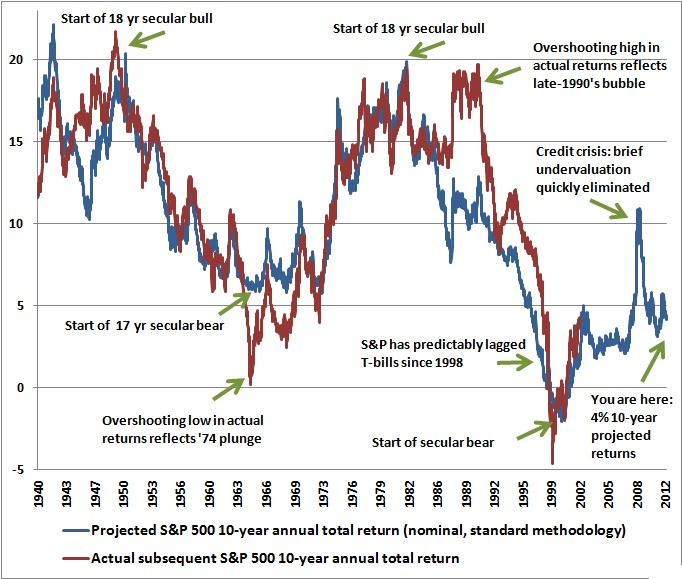Feeds:
Posts

## How to estimate the long-term return on stocks

There are a number of studies on the estimation of long-term returns to stocks. Ignoring the empirical research momentarily, the best explication of the estimation of long-term returns is by John Hussman in his article Valuations Matter. The logic is  straight forward.

According to Hussman, the long-term return on a security consists of two parts: income (from dividends or interest payments), and capital gains (from price changes). For any future stream of income, the higher the price you pay , the lower the annual rate of return you will earn:

Consider, for simplicity, a 30-year zero-coupon bond with a face value of \$100. If the bond is priced at a yield-to-maturity of 10%, it will cost you \$5.73 today. Over the coming 30 years, the price will advance to \$100, and your annualized return will be 10%. Just what you bargained for.

But what happens in the meantime? Suppose that over the first 10 years of your holding period, interest rates decline, and the yield-to-maturity on your bond falls to 7%. With 20 years remaining to maturity, the price of the bond will be \$25.84. Now here’s the crucial point. Even though the yield-to-maturity for the remaining life of the bond is just 7%, and the yield-to- maturity you bargained for when you bought the bond was only 10%, the return you have earned over the first 10 years is an impressive 16.26%!

By holding the security during a period when the yield-to-maturity is falling, you not only earn a return that is higher than the original yield to maturity, you earn a return that is dramatically higher than the future yield-to-maturity!

Now, the rest of the story. Over the remaining 20 years of the bond, you will not earn 16.26% annually, but 7% annually. If you do the math, you will find that over the entire 30 year holding period, you will have made — surprise — 10% annually. Just what you bargained for originally.

Here Hussman applies the same logic to the stock market:

For stocks, the “yield-to-maturity” comes from two components: income plus capital gain. The income component is simply the dividend yield. Assume initially that the dividend yield is held constant over time (we’ll relax this assumption in a moment). If the dividend yield (Dividend/Price) is constant, then by definition, prices must grow at exactly the same rate as dividends grow. By definition, when the dividend yield is unchanged between the date you buy stocks and the date you sell them, your total return equals the dividend yield (income) plus the growth rate of dividends (capital gain).

As a rule, a good estimate of the “yield-to-maturity” on stocks is the 6% long term growth rate plus the dividend yield. But remember, your actual return will only be equal to this value if the dividend yield stays constant over the period that you hold stocks. As we saw in our example, if the yield falls during the period you are holding stocks, your actual return will be even higher than the yield-to-maturity that you bargained for. On the other hand, if the yield on stocks rises over your holding period, your actual return will be even less than the yield-to-maturity you bargained for.

Historically, the dividend yield on stocks has averaged about 4%, and has fluctuated both above and below this 4% figure. As a result, the historical average return on stocks has typically been 6% + 4% = 10%. That’s precisely where that 10% “historical return” on stocks comes from.

Hussman provides the following equation to mathematically estimate the total return on stocks over any future time horizon (annualized):

(1+g)(Original Yield/Terminal Yield)1/N – 1 + (Original + Terminal)/2

Where Original Yield is the original dividend yield (in decimal form), Terminal Yield is the dividend yield expected at the end of the holding period, N is the holding period in years, and g is the growth rate of dividends over the holding period.

I find the logic appealing, but we should also consider how well the equation predicts the subsequent performance of the market. Here’s a chart from a recent Weekly Market Comment showing the projections for 10-year annual total returns on the S&P 500 versus actual subsequent 10-year total returns:Seems like a pretty good fit. The kicker: Hussman wrote his “Valuations Matter” article in June 1998, at which time he said of the market:

Currently, assuming dividend growth speeds up to a 6% rate and that the dividend yield is still just 1.4% in the future, the long term total return on stocks will be 7.4%. But here’s a more likely result: suppose the future dividend yield rises even a bit, even to just 2%. If that happens over the next 5 years, investors will earn a total return of zero over those 5 years. Over the next 10 years: just 4% annually. Over the next 20 years: 5.8% annually. Over the next 30 years: 6.4% annually. If the dividend yield rises to the historical average of 4% even 30 years from now, investors will have earned a total return of just 5% annually over that span. Consider that figure long and hard before trusting your retirement plans to a buy-and-hold approach in stocks.

It’s now 14 years since Hussman wrote the article. As difficult as it would been to believe it at the time, if anything, it seems at this point that Hussman was too optimistic.

Hussman’s rule of thumb seems like a sensible one:

You want to own stocks when the yield on stocks is high, or while favorable market action (interest rates, inflation, market breadth) are uniformly driving the yield downward. Beware when neither is true.

Tomorrow, I’ll show some estimates for the market as it stands now.

### One Response

1. […] Comments « How to estimate the long-term return on stocks […]

Like